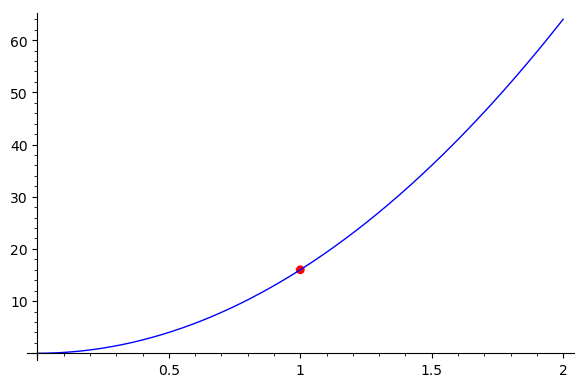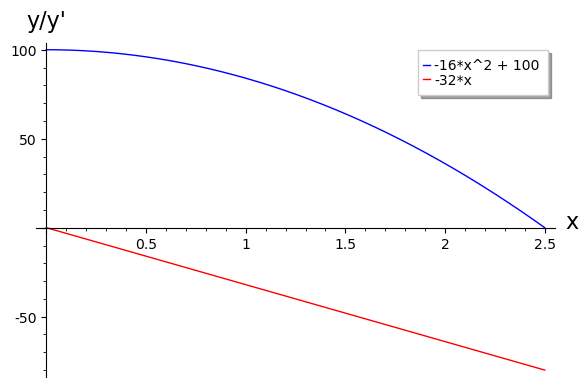CoCalc Public FilesLabs / Lab5 / Lab5.ipynb
Author: Kimberly Vargas Negrete
Views : 152
Compute Environment: Ubuntu 20.04 (Default)

## Curves and Lines

The rate of change of a function at a point has an important geometric meaning that you will explore in this section. To do this, you’ll need to be able to plot points, which is done with the command point.

Example 1. To plot a point at the coordinates (1,2), use the command point([1,2]). Notice that the coordinates are given as a list.

Example 2. To plot a larger red point at the coordinates (-3,5), use the command point([-3,5], color="red", size=40).

Exercise 1. Plot the function $h(x) = 16x^2$ for values of x between 0 and 5. Overlay the point (1,16) on the graph and make sure the point is visible.

In :
plot(16*x^2, (x, 0, 5)) + point([1,16], color="red", size=40)In :
plot(16*x^2, (x, 0, 2)) + point([1,16], color="red", size=40)In :
plot(16*x^2, (x, 0.5, 1.5)) + point([1,16], color="red", size=40)In [ ]:



Exercise 2. Create an animation that zooms in on this point by reducing the range of $x$. Use at least five different zoom levels. What do you observe?

In :
plots=[]
[1,0.8,0.6,0.4,0.2]
for h in [1,0.8,0.6,0.4,0.2]:
r=plot(16*x^2, (x,1-h,1+h)) + point([1,16], color="red", size=40)
plots.append(r)
a=animate (plots)
show(a)You should have noticed that as you zoom in on the curve, it starts to look like a straight line. (Some points on certain curves will not do this, which is an important observation but not very common in mathematical biology.) The question is what straight line it is.

To answer this question, we want to plot lines with slopes corresponding to the average rates of change we computed in the previous lab. Since we have a point and a slope, this is most easily done using the point-slope equation for a line passing through the point $(x_1,y_1)$. This equation is $y-y_1 = m(x-x_1)$. However, since Sage’s plot command requires everything other than y to be on the right-hand side of the equation, we have to rearrange it into $y = m(x - x_1) + y_1$.

Exercise 3. Create an interactive that plots the function f(x) = $16x^2$ and a line that passes through the point (1,16) and a second point on the function whose $x$-coordinate is set by the slider. Describe what happens as the movable point approaches the fixed one.

In :
var("x") f(x)=16*x^2 @interact def secline1(x2=(0,5,.2)):     m=((f(x2))-16)/(x2-1)     b=(-x2*m+f(x2))     i=plot(f(x),(x,0,5))+plot(m*x+b, (x,0,5))+ point([x2,f(x2)], color="green", size=40)     show(i)

In :
#as the slider is brought closer to the fixed line the difference in x value decreases until you get to 1. At this point the delta x value is zero and because of that the graph gives us an error.


Exercise 4. You can display a number on a plot using the text function. The syntax to display the value val at point pt on a plot is text(val, pt). (As in other cases, you can give the coordinates of pt as a list.) Modify your interactive so it computes and displays the slope of the line being plotted. HINT: Use + to overlay text on a plot.

In :
var("x") f(x)=16*x^2 @interact def secline1(x2=(0,5,.2)):     m=((f(x2))-16)/(x2-1)     b=(-x2*m+f(x2))     i=plot(f(x),(x,0,5))+plot(m*x+b, (x,0,5))+ point([x2,f(x2)], color="green", size=40)+text(m,[2,150])     show(i)


So far, we’ve been working with derivatives at single points. However, we can compute the derivative of a function at all points on the function. We then obtain a new function, $f'(x)$. Just like the number that gives the rate of change of a function at a single point, $f'(x)$ is termed the derivative of $f(x)$.

In Sagemath, derivatives are computed using the function diff. For example, to find the derivative of $e^{3x}$ with respect to $x$ and assign it to fp(x) enter fp(x)=diff(e^(3*x),x). As usual with an assignment, to print the result you will need to include an extra line where you print print(fp(x)) or show show(fp(x)) it. You can evaluate it for a particular $x$ value using the usual function evaluation notation fp(2) gives the value of the derivative at $x=2$.

Example 3. What is the derivative of $f(x) = 5x^{16}$ at $x = 1.5$?

In :
d(x)=diff(5*x^16,x)
show(d(x))

$80 \, x^{15}$
In :
d(1.5)

35031.5112304688

Exercise 5. Use Sagemath to find the derivatives of each of the following functions:

1. $f(x)=x^3$
2. $H(t)=200-16t^2$
3. $g(x)=\frac{x^3-1}{x^2+1}$
4. $M(t)=10e^{2t}$

Use show() to print out the formulas nicely. You may want to experiment with factor() and simplify() to see if you can get a nicer form for $g(x)$.

In :
#1
f1(x)=diff(x^3,x)
show(f1(x))

f1(1.5)

$3 \, x^{2}$
6.75000000000000
In :
#2
H(t)=diff(200-16*t^2)
show(H(t))


$-32 \, t$
In :
#3
var("X")
simplify(x^3-1)/(x^2+1)

(x^3 - 1)/(x^2 + 1)
In :
#3
g(x)=diff((x^3-1)/(x^2+1))
show(g(x))

$\frac{3 \, x^{2}}{x^{2} + 1} - \frac{2 \, {\left(x^{3} - 1\right)} x}{{\left(x^{2} + 1\right)}^{2}}$
In :
#4
M(t)=diff(10*e^(2*t))
show(M(t))

$20 \, e^{\left(2 \, t\right)}$

In Example 4 we start with a function $f(x)=x^3+2x^2-x+1$ and use Sagemath to find the derivative $f'(x)$, and then plot $f(X)$ and $f'(x)$ between $x=-3$ and $x=2$ with legend labels. Execute the two cells below to see what they produce.

Example 4.

In :
f(x)=x^3+2*x^2-x+1  ## Our function
fp(x)=diff(f(x),x)  ## fp(x) is the derivative
show("f(x)=",f(x))  ## show the formula for f(x) in a pretty form
show("f'(x)=",fp(x))## show the formula for f'(x) below it

$\verb|f(x)=| x^{3} + 2 \, x^{2} - x + 1$
$\verb|f'(x)=| 3 \, x^{2} + 4 \, x - 1$
In :
## Now we plot them and add legends
p1=plot(f(x),(x,-3,2),color='blue',legend_label=f(x))    ## generate the plot of f(x) from -3 to 2, store in p1
p2=plot(fp(x),(x,-3,2),color='red',legend_label=fp(x))   ## generate the plot of f'(x) from -3 to 2, store in p2
show(p1+p2,axes_labels=["x","y/y'"])   ## combine  the plots and add axes labelsExercise 6. Use the example above to create a function plot_f_fp(f,xmin,xmax) that takes as input a function f(x) and the range of x values for the plots and produces a plot with both $f(x)$ and $f'(x)$. Verify that it works correctly by checking that when you invoke it with plot_f_fp(f,-3,2) that it produces the same plot as in example 4, and that if you instead use

var('t'); H(t)=100-16*t^2; plot_f_fp(H,0,2.5)

you get a plot showing the height of a falling ball as on page 72 combined with a plot of the velocity of the ball which starts at 0 and goes linearly to -80 ft/sec.In :
def plot_f_fp(f,xmin,xmax):     fp(x)=diff(f(x),x)     show("f(x)=",f(x))     show("fprime(x)=",fp(x))     p1=plot(f(x),(x,xmin,xmax),color="blue",legend_label=f(x))     p2=plot(fp(x),(x,xmin,xmax),color="red",legend_label=fp(x))     show(p1+p2,axes_labels=["x","y/y"])

In :
plot_f_fp(f,-3,3)

$\verb|f(x)=| x^{3} + 2 \, x^{2} - x + 1$
$\verb|fprime(x)=| 3 \, x^{2} + 4 \, x - 1$In :
var('t') H(t)=100-16*t^2

In :
plot_f_fp(H,-3,3)

$\verb|f(x)=| -16 \, x^{2} + 100$
$\verb|fprime(x)=| -32 \, x$We know that the tangent line to a curve $y=f(x)$ at a point $a$ can be written down using the point slope form $y-y_0=m(x-x_0)$ where in this case the point $(x_0,y_0)=(a,f(a))$. We can rewrite that to get the formula for the tangent line as $y=f(a)+f'(a)(x-a)$.

Example 5. Use $f(x)=x^3+2x^2-x+1$ and draw a graph showing the function f(x) and the tangent line to the function at $a=3/4$. We will add a dot showing the point of tangency.

In :
f(x)=x^3+2*x^2-x+1  ## Our function
fp(x)=diff(f(x),x)  ## the derivative, f'
a=3/4
m=fp(a)             ## slope of the tangent line
t(x)=f(a)+m*(x-a)   ## formula for the tangent line
p1=plot(f(x),(x,-2,2),color='blue')      ## generate the plot of f(x) from -2 to 2, store in p1
p2=plot(t(x),(x,-2,2),color='red')       ## generate the plot of the tangent line from -2 to 2, store in p2
p3=point((a,f(a)),size=40,color='black') ## add a point, put it in p3
show(p1+p2+p3,axes_labels=["x","y"])     ## combine  the plots and add axes labelsExercise 7. Modify Example 5 to produce a plot showing the tangent line to $h(x)=\frac{e^x-e^{-x}}{e^x+e^{-x}}$ at $x=1$. Plot from $x=-3$ to $x=3$.

In :
f(x)=(e^x-e^-x)/(e^x+e^-x) fp(x)=diff(f(x),x) a=1 m=fp(a) t(x)=f(a)+m*(x-a) p1=plot(f(x),(x,-3,3),color='blue') p2=plot(t(x),(x,-3,3),color='red') p3=point((a,f(a)),size=40,color='black') show(p1+p2+p3,axes_labels=["x","y"])Exercise 8. Use diff to create an animation showing the tangent line to a function at different points, like the one at http://en.wikipedia.org/wiki/File:Graph_of_sliding_derivative_line.gif. (You don't need to make the line change color.)

In :
f(x)=(e^x-e^-x)/(e^x+e^-x) fp(x)=diff(f(x),x) plots=[] for a in srange(-3,3,.1):     m=fp(a)     t(x)=f(a)+m*(x-a)     p1=plot(f(x),(x,-3,3),color='pink',ymin=-1.5,ymax=1.5)     p2=plot(t(x),(x,-3,3),color='red',ymin=-1.5,ymax=1.5)     p3=point((a,f(a)),size=40,color='black')     p=p1+p2+p3     plots.append(p) a=animate(plots) show(a)

WARNING: Some output was deleted.
In [ ]: